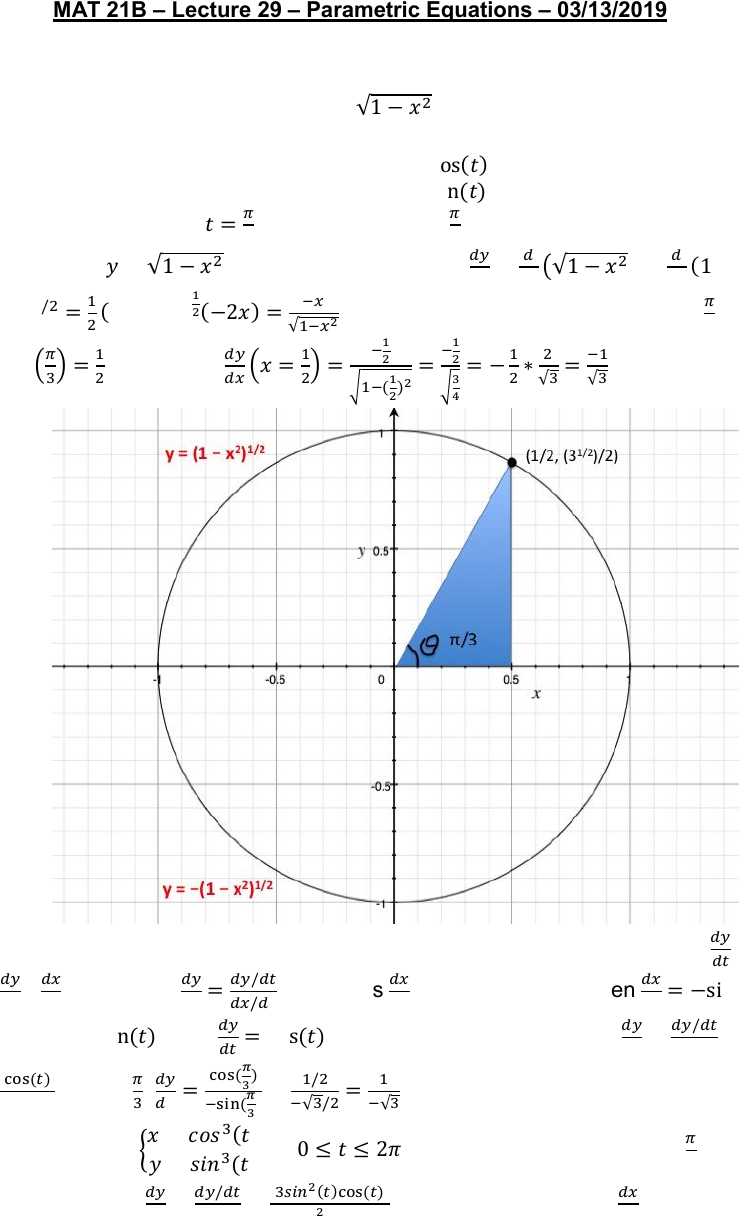Get 2 days of unlimited access
Class Notes (1,000,000)
US (420,000)
UC-Davis (9,000)
MAT (1,000)
MAT 21B (200)
Lecture 29

# MAT 21B Lecture Notes - Lecture 29: Unit Circle, Product Rule, Direct Comparison TestExamPremium

Department
Mathematics
Course Code
MAT 21B
Professor
L. Silverstein
Lecture
29

This preview shows page 1. to view the full 4 pages of the document.MAT 21B Lecture 29 Parametric Equations 03/13/2019
Suppose we have the unit circle of radius 1. In terms of x and y, the circle is
represented by or  . However, we can represent that
same circle by first introducing a parameter, t.
A parameterization for the unit circle is  
   for  . Find the slope
of the tangent line at
. Since    at  
, then we choose the positive
solution of . Next, take its derivative 





. The x-value that corresponds to  
is  

. Therefore, 





.
There is an alternative method to solving this problem. By the chain rule, 


 
. Therefore, 
 
 as long as 
 . If  , then 
 
and if   , then 
 . Applying the formula, we get 
 


. At  
, 



.
Example: Let 
 for . What is the slope when  
?
First, compute 
 
 
. Find the points where 
 . We have
   or  or
 or . On   , the values of t that satisfy these
###### You're Reading a Preview

Unlock to view full version

Subscribers Only